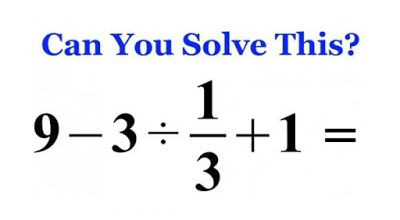### Solve This! A Quick Puzzle to Start Your Week

I think this is the simplest maths equation you would ever come across. Give it a shot and don't beat yourself up if you don't get it. Better to try and fail than never to try at all. Good luck!#### Related Posts

###### Nigeria Does not Determine WAEC Timetable - PTF Cordinator

9
Follow
1 year ago
• sannmotu: year the ans is 1
1 year ago
• ROBERT: The answer is 3.
1 year ago
• Perseverance: 0 is d ans
1 year ago
• Eniola Oladokun: The correct answer from here to U.S.A is nothing,but 1.
1 year ago
• MASTER: The answer is 3
1 year ago
•      1 year ago
• Mespu: 3 is the answer
1 year ago
• NAZIFA IBRAHIM USMAN: The answer is 3
1 year ago
• Prosper: The answer is nineteen
1 year ago
• Kaydan: 1
1 year ago
• abisco: Using bodmas

9 - (3 x3) + 1 [ convert division to multiplication]
9 - 9 + 1
0 + 1
= +1
=1
1 year ago
• PRINCETEC: 1 SHOULD BE THE ANSWER WHEN USE BODMAS
1 year ago
• Perseverance: the answer is 3
2 months ago
•      2 months ago
• Amanda: 7
2 months ago
Bodmas
3*3=9
9-9 +1 =1
2 months ago
•      2 months ago
• Emmable: The answer is 1
2 months ago
• Best Brain: 9-3÷1/3+1
6÷1/3+1
6÷2/3
2÷2= 1
2 months ago
• HARUNA: -1 is the answer
2 months ago
• Lorlah™️: 9-3÷1/3+1=-1
2 months ago
•      2 months ago
• Aleeyou: 3
2 months ago
• Damilola: the correct answer is 1 using bodmas
2 months ago
• Abiodun019: Using BODMAS.....
1÷3=0.3333.....
3÷0.33333=9.0
(9)-(3)÷(1÷3)+(1)=?
(9)-(3)÷(0.33333)+1=?
(9)-(9.0)=0
2 months ago
• davison20: The answer is 1
From bodmas rule
Division first
3÷1/3=3*3=9
From the first equation we now have
9-9+1=1
2 months ago
• Martin edu: 4.5
2 months ago
• lescott619: The answer is 1
2 months ago
•      2 months ago
•      2 months ago
•      2 months ago
• PETER: The answer is 1
2 months ago
• Ignatius: answer is -1 (minus one)
2 months ago
• Osayuki: the final answer is 1
2 months ago
2 months ago
• sir nature: 9-3/1/3+1=3
2 months ago
2 months ago
• Chinemerem: 9-3÷1/3+1=
solution:
using bodmas
3÷1/3 =3×3/1 = 9
9+1 = 10
9-10 = -1
2 months ago
• Muizdino: The answer is -1
2 months ago
• maganazuru: the answer is 1
2 months ago
2 months ago
• dami: 1
2 months ago
• Purity: 1
2 months ago
• Carrie: 9-3divide 1/3 +1=? using (BODMAS), we go for division first;we have since any number have an invisible one (1) under it, so we have 3/1 divide 1/3 =0,we bring down 9-0+1=10.Answer =10.
2 months ago
• Bree: using bodmas
9-3*3after cross multiplying
=9-9+1
=0+1=1ans
2 months ago
• Great: 9_3÷1\3+1=6×3\1+1=18+1=19
2 months ago
• Edna: 1
2 months ago
•      1 month ago
• Rosie: 1
1 month ago
Follow
• Abayomi David Omishola: 9-3×3-1+1
multiply the numbers
9-9+1
eliminate the opposite
=1.1 year ago
•      2 months ago
• samcooltobi34: 9-3÷1/3+1
9-3*3/1+1
9-9/1+1
=1
2 months ago
• Pet: the correct answer is 1
2 months ago
• Pet:
2 months ago
•      2 months ago
• donv101: -1
2 months ago
• sir nature: The answer is 1
2 months ago
• Johnsane: the answer is 1
1 month ago
•      1 month ago
the ans is 9.00 to 2dp
Follow
• sheriff: the ans. is 3
2 months ago
1 is the correct ans
Follow
• Pet:
2 months ago
1/2
Follow
• Euler: lol... 9-3÷1/3+1
= 9-(3÷1/3)+1
= 9-(3×3/1)+1
= 9-(9)+1
= 9-9+1
= 9-8
=1
1 year ago
Follow
-1
Follow
1
Follow
Follow
1
Follow
i think the answer is +1
Follow
Follow
Follow
Follow
explanation: by using bodmas we will firstly turn the division sign to multiplication then multiply the 3 by 3 to give 9 add 1 to it and the subtract it by 9
Follow

#### Quick Questions

• 3 Answers   ·   1 hour ago
• 2 Answers   ·   1 hour ago
• 2 Answers   ·   1 hour ago

#### Post Comment

Please don't post or ask to join a "Group" or "Whatsapp Group" as a comment. It will be deleted. To join or start a group, please click here

1 Members
4 Members
• 21st
Jul 2020
##### Marketplace
• Here are the things students around you have to sell;
• ###### Samsung galaxy Grand prime+
₦36,000   Used
• ###### Digital SLR Camera Sony HX 200V
₦70,000   OFFAPOLY   Used